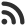Feed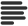Articles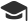Tutorials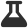CodingLab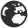CommunityLeaderboardFounder & Programmer at HackersFriend Updated Nov. 13, 2019, 10:43 p.m. ⋅ 118 views

# Implement a Dictionary using Trie

In this article I'll implement a dictionary using Trie Data Structure. We'll discusss implementation of a dictionary using Trie (memory optimization using hash-map).

Let's take a problem and that requires dictionary to solve, this way we'll understand the in better way.

Problem

There are some name of students and we have their corresponding marks. Now the problem is to tell the marks of a student when his/her name is given as input.

Approach

There are other ways to solve this problem also but here, we'll store students and their marks in a dictionary.

Student name will be the key and his/her mark will be value.

We'll implement this dictionary using Trie. Trick is to add an extra data to the node of trie. i.e the mark of student. Whenever a new query is made, we'll search for that name of student in the Trie and if it is found, we'll return the value we are storing with it. Otherwise we'll return -1.

Implementation

``````// C++ implementation of the approach

#include <bits/stdc++.h>
using namespace std;

// Structure for Trie

struct Trie {
bool isEndOfName;
unordered_map<char, Trie*> map;
int marks;
};

// Function to create a new Trie node
Trie* getNewTrieNode()
{
Trie* node = new Trie;
node->isEndOfName = false;
return node;
}

// Function to insert a student with its marks
// in the dictionary built using a Trie

void insert(Trie*& root, const string& str,
const int& marks)
{

// If root is null
if (root == NULL)
root = getNewTrieNode();

Trie* temp = root;

for (int i = 0; i < str.length(); i++) {
char x = str[i];

// Make a new node if there is no path
if (temp->map.find(x) == temp->map.end())
temp->map[x] = getNewTrieNode();

temp = temp->map[x];
}

// Mark end of Name and store the meaning
temp->isEndOfName = true;
temp->marks = marks;
}

// Function to search a student in the Trie and return its marks if it exists

string getMarks(Trie* root, const string& student)
{

// If root is null i.e. the dictionary is empty
if (root == NULL)
return "-1";

Trie* temp = root;

// Search a student in the Trie

for (int i = 0; i < student.length(); i++) {
temp = temp->map[student[i]];
if (temp == NULL)
return "-1";
}

// If it is the end of a valid name stored then return its marks

if (temp->isEndOfName)
return temp->marks;
return "-1";
}

// Main function

int main()
{
Trie* root = NULL;

// Build the dictionary
insert(root, "Alice", 80);
insert(root, "Pooja", 85);
insert(root, "Ramesh", 83);
insert(root, "Mark", 88);

string str = "Mark";
cout << getMarks(root, str);

return 0;
}
``````

Output

``88``

 arrow_upward UpvoteComment arrow_downward downvote

Events

Nov. 28, 2018, 5:30 p.m.

Python from zero to hero

place Delhi

Aug. 13, 2018, 5:30 p.m.

Python from zero to hero

place Bangalore ( HackersFriend office BTM Layout)